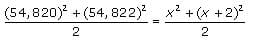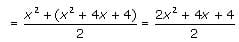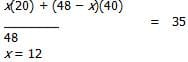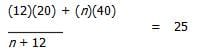# Test: Mean

## 10 Questions MCQ Test Practice Questions for GMAT | Test: Mean

Description
Attempt Test: Mean | 10 questions in 20 minutes | Mock test for GMAT preparation | Free important questions MCQ to study Practice Questions for GMAT for GMAT Exam | Download free PDF with solutions
QUESTION: 1

### During 2005, a company produced an average of 2,000 products per month. How many products will thecompany need to produce from 2006 through 2008 in order to increase its monthly average for the periodfrom 2005 through 2008 by 200% over its 2005 average?

Solution:

A 200% increase over 2,000 products per month would be 6,000 products per month.  (Recall that 100% = 2,000, 200% = 4,000, and "200% over" means 4,000 + 2,000 = 6,000.) In order to average 6,000 products per month over the 4 year period from 2005 through 2008, the company would need to produce 6,000 products per month × 12 months × 4 years = 288,000 total products during that period. We are told that during 2005 the company averaged 2,000 products per month. Thus, it produced 2,000 × 12 = 24,000 products during 2005. This means that from 2006 to 2008, the company will need to produce an additional 264,000 products (288,000 – 24,000). The correct answer is D.

QUESTION: 2

Solution:
QUESTION: 3

### A certain portfolio consisted of 5 stocks, priced at \$20, \$35, \$40, \$45, and \$70, respectively. On a givenday, the price of one stock increased by 15%, while the price of another stock decreased by 35% and theprices of the remaining three remained constant. If the average price of a stock in the portfolio rose byapproximately 2%, which of the following could be the prices of the shares that remained constant?

Solution:
QUESTION: 4

If John makes a contribution to a charity fund at school, the average contribution size will increase by50%, reaching \$75 per person. If there were 5 other contributions made before John’s, what is the size ofhis donation?

Solution:
QUESTION: 5

What is the minimum percentage increase in the mean of set X {-4, -1, 0, 6, 9} if its two smallest elementsare replaced with two different primes?

Solution:
QUESTION: 6

Which of the following series of numbers, if added to the set {1, 6, 11, 16, 21}, will not change the set’smean?​
I. 1.5, 7.11 and 16.89
II. 5.36, 10.7 and 13.24
III. -21.52, 23.3, 31.22

Solution:
QUESTION: 7

If numbers N and K are added to set X {2, 8, 10, 12}, its mean will increase by 25%. What is the value of N2 + 2NK + K2 ?

Solution:
QUESTION: 8

The mean of (54,820)2 and (54,822)2 =

Solution:

We can simplify this problem by using variables instead of numbers. x = 54,820,
x + 2 = 54,822. The average of (54,820)2 and (54,822)2= x2+2x+2

Now, factor x2 + 2x +2. This equals x2 + 2x +1 + 1, which equals (x + 1)2 + 1.
Substitute our original number back in for x as follows:
(x + 1)2 + 1 = (54,820 + 1)2 + 1 = (54,821)2 + 1.

QUESTION: 9

Set S consists of integers 7, 8, 10, 12, and 13. If integer n is included in the set, the average (arithmetic
mean) of set S will increase by 20%. What is the value of integer n?

Solution:

First, let’s use the average formula to find the current mean of set S: Current mean of set S = (sum of the terms)/(number of terms): (sum of the terms) = (7 + 8 + 10 + 12 + 13) = 50    (number of terms) = 5    50/5 = 10
Mean of set S after integer n is added = 10 × 1.2 = 12         Next, we can use the new average to find the sum of the elements in the new set and compute the value of integer n. Just make sure that you remember that after integer n is added to the set, it will contain 6 rather than 5 elements.     Sum of all elements in the new set = (average) × (number of terms) = 12 × 6 = 72     Value of integer n = sum of all elements in the new set – sum of all elements in the original set = 72 – 50 = 22     The correct answer is D.

QUESTION: 10

A convenience store currently stocks 48 bottles of mineral water. The bottles have two sizes of either 20 or 40 ounces each. The average volume per bottle the store currently has in stock is 35 ounces. How many 40 ounce bottles must be sold for the average volume per bottle to be reduced to 25 ounces if no 20 ounce bottles are sold?

Solution:

Let x = the number of 20 oz. bottles     48 – x = the number of 40 oz. bottles     The average volume of the 48 bottles in stock can be calculated as a weighted average:Therefore there are 12 twenty oz. bottles and 48 – 12 = 36 forty oz. bottles in stock. If no twenty oz. bottles are to be sold, we can calculate the number of forty oz. bottles it would take to yield an average volume of 25 oz:
Let n = number of 40 oz. bottles(12)(20) + 40n = 25n + (12)(25)
15n = (12)(25) – (12)(20)
15n = (12)(25 – 20)
15n = (12)(5)
15n = 60
n = 4
Since it would take 4  forty oz. bottles along with 12 twenty oz. bottles to yield an average volume of 25 oz, 36 – 4 = 32 forty oz. bottles must be sold. The correct answer is D.Use Code STAYHOME200 and get INR 200 additional OFF Use Coupon Code